# D2q9 pf velocity debug autosym

## Description

The 'd2q9_pf_cm' model is a multiphase 2D (planar) lattice Boltzmann model for the simulation of incompressible, immiscible fluids (at both high and low density ratios).

The base implementation uses an incompressible, velocity based LBM for capturing the hydrodynamics of the flow and solves the conservative phase field equation for the interfacial dynamics. To enhance stability, a Multiple-Relaxation-Time and Cascaded collision operator are used.

The MRT model has 2 options at compile time:

• GF: Guo Forcing;

This is using a higher order Forcing scheme from the work of Guo et al. (2002) for the hydrodynamics

• RT: Ren Temporal

This is using the Temporal term included in the phase field equilibrium distribution function by Ren et al. (2016)

Publications: " Improved locality of the phase-field lattice Boltzmann model for immiscible fluids at high density ratios " Authors: A. Fakhari, T. Mitchell, C. Leonardi, D. Bolster (2017) DOI: 10.1103/PhysRevE.96.053301

Boundary Conditions options at compile time: * Outflow:

  This is used for outflow boundaries, it is made as an
option as it requires additional fields for calculations
so results in a slower code.

• autosym:

Allows symmetry node type flags introduced in v6.2

Updates: 16/08/2018: Cascaded (CLBM) collision scheme is introduced for both hydrodynamics and phase-field.

## Details

Model description files files for this model: Dynamics.c Dynamics.R

### Zonal Settings

Name Comment
PhaseField_init Initial/Inflow PhaseField distribution
VelocityX inlet/outlet/init velocity
VelocityY inlet/outlet/init velocity
Pressure inlet/outlet/init density
GravitationX applied (rho)*GravitationX
GravitationY applied (rho)*GravitationY
PressureLossInObj Weight of [pressure loss] in objective
OutletFluxInObj Weight of [pressure loss] in objective
InletFluxInObj Weight of [pressure loss] in objective
TotalDensityInObj Weight of [Mass conservation check] in objective
RTIBubbleInObj Weight of [Bubble Tracker] in objective
RTISpikeInObj Weight of [Spike Tracker] in objective
NMovingWallForceInObj Weight of [force exerted on the N Moving Wall] in objective
NMovingWallPowerInObj Weight of [implented: Vx* incoming momentum (precollision)] in objective
MomentumXInObj Weight of [Total momentum in the domain] in objective
MomentumYInObj Weight of [Total momentum in the domain] in objective
MomentumX_afterColInObj Weight of [Total momentum in the domain] in objective
MomentumY_afterColInObj Weight of [Total momentum in the domain] in objective
F_pressureXInObj Weight of [Pressure force X] in objective
F_pressureYInObj Weight of [Pressure force Y] in objective
F_bodyXInObj Weight of [Body force X] in objective
F_bodyYInObj Weight of [Body force Y] in objective
F_surf_tensionXInObj Weight of [Surface tension force X] in objective
F_surf_tensionYInObj Weight of [Surface tension force Y] in objective
F_muXInObj Weight of [Viscous tension force X] in objective
F_muYInObj Weight of [Viscous tension force Y] in objective
F_total_hydroXInObj Weight of [Total hydrodynamic force X] in objective
F_total_hydroYInObj Weight of [Total hydrodynamic force Y] in objective
F_phiXInObj Weight of [Forcing term for interface tracking X] in objective
F_phiYInObj Weight of [Forcing term for interface tracking Y] in objective
FDragInObj Weight of [Force exerted on body in X-direction] in objective
FLiftInObj Weight of [Force exerted on body in Y-direction] in objective
FTotalInObj Weight of [Force exerted on body in X+Y -direction] in objective

### Global Settings

Name Derived Comment
Period Number of cells per cos wave
Perturbation Size of wave perturbation, Perturbation Period
MidPoint height of RTI centerline
Radius Radius of diffuse interface circle
CenterX Circle center x-coord
CenterY Circle Center y-coord
BubbleType Drop/bubble
Density_h High density fluid
Density_l Low density fluid
PhaseField_h PhaseField in high density fluid
PhaseField_l PhaseField in low density fluid
W Anti-diffusivity coeff (phase interfacial thickness)
omega_phi 1.0/(3*M+0.5) one over relaxation time (phase field)
M Mobility
sigma surface tension
ContactAngle Contact angle in degrees - in reference to the high density fluid
radAngle ContactAngle*3.1415926535897/180 Conversion to rads for calcs
tau_l (3*Viscosity_l) relaxation time (low density fluid)
tau_h (3*Viscosity_h) relaxation time (high density fluid)
Viscosity_l kinematic viscosity
Viscosity_h kinematic viscosity
omega_bulk 1.0/(3*bulk_visc+0.5) inverse of bulk relaxation time
bulk_visc bulk viscosity
BuoyancyX applied (rho-rho_h)*BuoyancyX
BuoyancyY applied (rho-rho_h)*BuoyancyY
fixedIterator fixed iterator for velocity calculation
Threshold Parameters threshold

### Exported Quantities (VTK, etc)

Name Unit Comment
Rho kg/m3 Rho
PhaseField 1 PhaseField
U m/s U
NormalizedPressure Pa NormalizedPressure
Pressure Pa Pressure
Normal 1 Normal

#### Exported Global Integrals (CSV, etc)

Name Unit Comment
RTIBubble 1 Bubble Tracker
RTISpike 1 Spike Tracker
PressureLoss 1mPa pressure loss
OutletFlux 1m2/s pressure loss
InletFlux 1m2/s pressure loss
TotalDensity 1kg/m3 Mass conservation check
NMovingWallForce 1 force exerted on the N Moving Wall
NMovingWallPower 1 implented: Vx* incoming momentum (precollision)
MomentumX  Total momentum in the domain
MomentumY  Total momentum in the domain
MomentumX_afterCol  Total momentum in the domain
MomentumY_afterCol  Total momentum in the domain
F_pressureX  Pressure force X
F_pressureY  Pressure force Y
F_bodyX  Body force X
F_bodyY  Body force Y
F_surf_tensionX  Surface tension force X
F_surf_tensionY  Surface tension force Y
F_muX  Viscous tension force X
F_muY  Viscous tension force Y
F_total_hydroX  Total hydrodynamic force X
F_total_hydroY  Total hydrodynamic force Y
F_phiX  Forcing term for interface tracking X
F_phiY  Forcing term for interface tracking Y
FDrag N Force exerted on body in X-direction
FLift N Force exerted on body in Y-direction
FTotal N Force exerted on body in X+Y -direction
Objective 1 Objective function

### Node Types

Group Types
BODY Body
BOUNDARY Wall, Solid, WVelocity, WPressure, WPressureL, EPressure, EVelocity, MovingWall_N, MovingWall_S, NVelocity
COLLISION BGK, MRT
DESIGNSPACE DesignSpace
NONE None
SETTINGZONE DefaultZone
SYMX SymmetryX_plus, SymmetryX_minus
SYMY SymmetryY_plus, SymmetryY_minus

### Solved fields

Name Access pattern Comment
g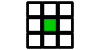g
g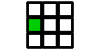g
g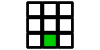g
gg
g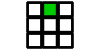g
g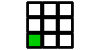g
g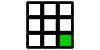g
g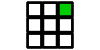g
g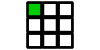g
hh
hh
hh
hh
hh
hh
hh
hh
hh
nw_xphase field normal at the wall in x direction, pointing into fluid
nw_ynw_y
UU
VV
PhaseF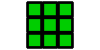PhaseF

### Densities - default accessors

Name Field Pulling field from Comment
g gg
g gg
g gg
g gg
g gg
g gg
g gg
g gg
g gg
h hh
h hh
h hh
h hh
h hh
h hh
h hh
h hh
h hh
nw_x nw_xphase field normal at the wall in x direction, pointing into fluid
nw_y nw_ynw_y
U UU
V VV

### Action stages

Name Main procedure Preloaded densities Pushed fields
PhaseInit Init_phase none PhaseF
WallInit Init_wallNorm none nw_x, nw_y
BaseInit Init_distributions none g, g, g, g, g, g, g, g, g, h, h, h, h, h, h, h, h, h, U, V
BaseIter calcHydroIter g, g, g, g, g, g, g, g, g, h, h, h, h, h, h, h, h, h, nw_x, nw_y, U, V g, g, g, g, g, g, g, g, g, h, h, h, h, h, h, h, h, h, nw_x, nw_y, U, V
PhaseIter calcPhaseFIter g, g, g, g, g, g, g, g, g, h, h, h, h, h, h, h, h, h, nw_x, nw_y, U, V PhaseF
WallIter calcWallPhaseIter nw_x, nw_y PhaseF

### Actions

Name Stages
Iteration BaseIter, PhaseIter, WallIter
Init PhaseInit, WallInit, WallIter, BaseInit